﻿ 疾病在食饵中进行传播的无差别捕食的捕食–食饵系统的定性分析 Qualitative Analysis of a Predator-Prey System with Undifferentiated Predation and Disease Is Spread in the Bait

Hans Journal of Computational Biology
Vol.07 No.04(2017), Article ID:24403,7 pages
10.12677/HJCB.2017.74005

Qualitative Analysis of a Predator-Prey System with Undifferentiated Predation and Disease Is Spread in the Bait

Hanchuan Chen, Yongjin Xian

Dalian Jiaotong University, Dalian Liaoning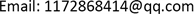Received: Mar. 27th, 2018; accepted: Apr. 11th, 2018; published: Apr. 18th, 2018ABSTRACT

In this paper, a predator-prey system is studied for the propagation of a class of diseases in the prey. The prey is restricted by density, and the predator does not discriminate whether the food is infected or not. This paper discusses the condition of the boundary equilibrium points of the model, and proves the local stability of the system according to the Routh-Hurwitz criterion by the Jacobian matrix. Using Bendixson-Dulac criterion, the ultimate proof system does not exist all the closed orbits in G. Thus the zero solution of the limit system is global stable. The global asymptotic stability of the original system is obtained by the limit system theory. Finally, the existence of the positive equilibrium and the limit cycle of the limit system are discussed.

Keywords:Predator-Prey System, Limit System, Stability, Limit Cycle1. 引言

$\left\{\begin{array}{l}\frac{\text{d}x}{\text{d}t}=x\left({a}_{1}-{b}_{1}x-{c}_{1}y\right)\\ \frac{\text{d}y}{\text{d}t}=y\left({a}_{2}-{b}_{2}y+{c}_{2}x\right)\end{array}$

$\left\{\begin{array}{l}\frac{\text{d}s}{\text{d}t}=\left(b-\frac{\theta rH}{K}\right)H-\left(d+\frac{\left(1-\theta \right)rH}{K}\right)s-\beta si\\ \frac{\text{d}i}{\text{d}t}=\beta si-\left(d+\frac{\left(1-\theta \right)rH}{K}\right)i-\frac{miy}{\alpha +i}\\ \frac{\text{d}y}{\text{d}t}=\left(\frac{\epsilon mi}{\alpha +i}-e\right)y\end{array}$ (1.1)

2. 模型改进

$\left\{\begin{array}{l}\frac{\text{d}s}{\text{d}t}=\left(b-\frac{\theta rH}{K}\right)H-\left(d+\frac{\left(1-\theta \right)rH}{K}\right)s-\beta si-\frac{msy}{\alpha +H}\\ \frac{\text{d}i}{\text{d}t}=\beta si-\left(d+\frac{\left(1-\theta \right)rH}{K}\right)i-\frac{miy}{\alpha +H}\\ \frac{\text{d}y}{\text{d}t}=\left(\frac{\epsilon mH}{\alpha +H}-e\right)y\end{array}$ (2.1)

$1-\frac{H}{K}\ge 0$ ，可得 $H\le K$ ，即 $s\left(t\right)+i\left(t\right)\le K$$\forall t\ge 0$ ，有 $s\left(t\right)\ge s\left(0\right)\ge 0$ ；同理， $i\left(t\right)\ge 0,y\left(t\right)\ge 0$ 。即可以得到 $G=\left\{\left(s,i,y\right):s+i\le K,s\ge 0,i\ge 0,y\ge 0\right\}$ 是方程组(2.1)的正不变集。

3. 平衡点及稳定性

3.1. 平衡点

$K\beta +r\theta -b>0$ 时，系统(2.1)存在边界平衡点 ${E}_{2}$ 。当 $i=0$ 时，系统将退化为一般的捕食–食饵系统，本文将不做讨论。

3.2. 局部稳定性

${J}_{{E}_{1}}=\left(\begin{array}{ccc}-r& d-r\theta -K\beta & -\frac{mK}{\alpha +K}\\ 0& K\beta -\left(d+\left(1-\theta \right)r\right)& 0\\ 0& 0& \frac{\epsilon mK}{\alpha +K}-e\end{array}\right)$ (3.1)

${J}_{{E}_{2}}=\left(\begin{array}{ccc}-\left(\theta r+\frac{\left(1-\theta \right)r{s}_{2}}{K}+\beta {i}_{2}\right)& b-2r\theta -\frac{\left(1-\theta \right)r{s}_{2}}{K}-\beta {s}_{2}& -\frac{m{s}_{2}}{\alpha +K}\\ \beta {i}_{2}-\frac{\left(1-\theta \right)r{i}_{2}}{K}& \beta {s}_{2}-d-\left(1-\theta \right)r-\frac{\left(1-\theta \right)r}{K}& -\frac{m{i}_{2}}{\alpha +K}\\ 0& 0& \frac{\epsilon mK}{\alpha +K}-e\end{array}\right)$ (3.2)

${R}_{1}>1$${R}_{2}<1$ 时，由Routh-Hurwitz判据可知 $\frac{\epsilon mK}{\alpha +K}-e<0$ ，考察以下矩阵：

${\stackrel{˜}{J}}_{{E}_{2}}=\left(\begin{array}{cc}-\left(\theta r+\frac{\left(1-\theta \right)r{s}_{2}}{K}+\beta {i}_{2}\right)& b-2r\theta -\frac{\left(1-\theta \right)r{s}_{2}}{K}-\beta {s}_{2}\\ \beta {i}_{2}-\frac{\left(1-\theta \right)r{i}_{2}}{K}& \beta {s}_{2}-d-\left(1-\theta \right)r-\frac{\left(1-\theta \right)r}{K}\end{array}\right)$ (3.3)

3.3. 全局稳定性

$i\ge C{\text{e}}^{\left(K\beta -\left(1-\theta \right)r-d\right)t}\text{ }$ (3.4)

$t\to +\infty$ 时， ${\text{e}}^{\left(K\beta -\left(1-\theta \right)r-d\right)t}\to 0$ ；由 $i\ge 0$ 和式(3.4)可知 $i=0$ ，即

$M=\left\{\left(s,i,y\right):\stackrel{˙}{V}=0\right\}=\left\{i=0\right\}$ (3.5)

$\left\{\begin{array}{l}\frac{\text{d}s}{\text{d}t}=rs\left(1-\frac{s}{K}\right)-\frac{msy}{\alpha +s}\\ \frac{\text{d}y}{\text{d}t}=\left(\frac{\epsilon ms}{\alpha +s}-e\right)y\end{array}$ (3.6)

$\left\{\begin{array}{l}\frac{\text{d}H}{\text{d}t}=rH\left(1-\frac{H}{K}\right)\\ \frac{\text{d}i}{\text{d}t}=\beta si-\left(d+\frac{\left(1-\theta \right)rH}{K}\right)i\end{array}$ (3.7)

$\left\{\begin{array}{l}\frac{\text{d}H}{\text{d}t}=rH\left(1-\frac{H}{K}\right)\\ \frac{\text{d}x}{\text{d}t}=-\left(d+r\right)x+\left(\beta +\frac{rH}{K}\right)Hx-\beta H{x}^{2}\end{array}$ (3.8)

${R}_{1}>1$ 且t充分大时，极限系统(3.7)在N中的解以 $\frac{K\beta +\theta r-b}{K\beta }$ 为极限。由此可知系统(3.8)的平衡点 $\left(K,\frac{K\beta +\theta \text{ }r-b}{K\beta }\right)$ 在N中全局渐近稳定，进而有等价系统(3.7)的平衡点 ${W}_{2}\left(K,{i}_{2}\right)$ 在G内全局渐近稳定。

$\left\{\begin{array}{l}\frac{\text{d}H}{\text{d}t}=rH\left(1-\frac{H}{K}\right)-\frac{mHy}{\alpha +H}\\ \frac{\text{d}i}{\text{d}t}=\beta si-\left(d+\frac{\left(1-\theta \right)rH}{K}\right)i-\frac{miy}{\alpha +H}\\ \frac{\text{d}y}{\text{d}t}=\left(\frac{\epsilon mH}{\alpha +H}-e\right)y\end{array}$ (3.9)

$\left\{\begin{array}{l}\frac{\text{d}H}{\text{d}t}=rH\left(1-\frac{H}{K}\right)-\frac{mHy}{\alpha +H}\\ \frac{\text{d}i}{\text{d}t}=\beta si-\left(d+\frac{\left(1-\theta \right)rH}{K}\right)i-\frac{miy}{\alpha +H}\end{array}$ (3.10)

3.4. 正平衡点及极限环

$\left\{\begin{array}{l}\frac{\text{d}H}{\text{d}t}=rH\left(1-\frac{H}{K}\right)-\frac{mHy}{\alpha +H}\\ \frac{\text{d}y}{\text{d}t}=\left(\frac{\epsilon mH}{\alpha +H}-e\right)y\end{array}$ (3.11)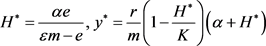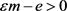，且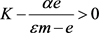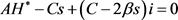(3.12)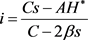(3.13)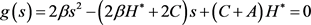(3.14)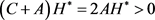(3.15)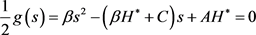(3.16)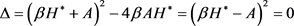，即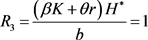时，方程(3.16)存在唯一正根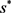；此时，系统(2.1)有唯一的正平衡点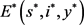，其中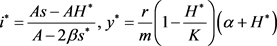。由定理3.1的证明过程可知，(3.17)

4. 结论

 赵姣, 魏春金, 王宝童, 张树文. 疾病在食饵中传播的捕食–食饵模型[J]. 生物数学学报, 2015(4): 709-713.

 Lasalle, J.P. (1968) Stability Theory for Ordinary Differential Equations. Journal of Differential Equations, 4, 57-65. https://doi.org/10.1016/0022-0396(68)90048-X

 陈兰荪, 井竹君. 捕食者–食饵相互作用中微分方程的极限环存在性和唯一性[J]. 科学通报, 1984, 29(9): 521-523.

 马知恩, 周义仓, 李承治. 常微分方程定性与稳定性方法[M]. 第二版. 北京: 科学出版社, 2015.